Main Content

# Parking Valet Using Nonlinear Model Predictive Control

This example shows how to generate a reference trajectory and track the trajectory for a parking valet using nonlinear model predictive control (NLMPC).

### Parking Garage

In this example, the parking garage contains an ego vehicle and eight static obstacles. The obstacles are given by six parked vehicles, a reserved parking area, and the garage border. The goal of the ego vehicle is to park at a target pose without colliding with any of the obstacles. The reference point of the ego pose is located at the center of the rear axle.

Define the parameters of the ego vehicle.

```vdims = vehicleDimensions; egoWheelbase = vdims.Wheelbase; distToCenter = 0.5*egoWheelbase;```

Specify the initial ego vehicle pose.

```% Ego initial pose: x(m), y(m) and yaw angle (rad) egoInitialPose = [4,12,0];```

Define the target pose for the ego vehicle. In this example, there are two possible parking directions. To park facing north, set `parkNorth` to `true`. To park facing south, set `parkNorth` to `false`.

```parkNorth = true; if parkNorth egoTargetPose = [36,45,pi/2]; else egoTargetPose = [27.2,4.7,-pi/2]; end```

The `helperSLCreateCostmap` function creates a static map of the parking lot that contains information about stationary obstacles, road markings, and parked cars. For more details, see the Automated Parking Valet in Simulink (Automated Driving Toolbox) example.

```costmap = helperSLCreateCostmap(); centerToFront = distToCenter; centerToRear = distToCenter; helperSLCreateUtilityBus; costmapStruct = helperSLCreateUtilityStruct(costmap);```

Visualize the parking environment. Use a sample time of `0.1` for the visualizer.

```Tv = 0.1; helperSLVisualizeParkingValet(egoInitialPose, 0, costmapStruct);```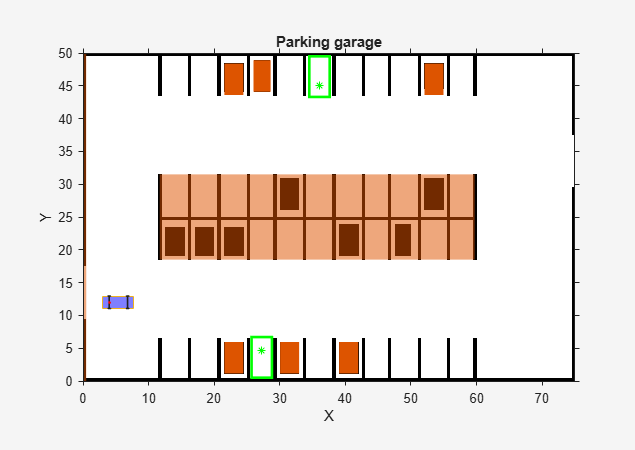The six parked vehicles are orange boxes on the top and bottom of the figure. The middle area represents the reserved parking area. The left border of the garage is also modeled as a static obstacle. The ego vehicle in blue has two axles and four wheels. The two green boxes represent the target parking spots for the ego vehicle, with the top spot facing north.

### Generate a Trajectory Using Nonlinear Model Predictive Controller

In this example, a kinematic bicycle model with front steering angle is used. The motion of the ego vehicle can be described by the following equations.

`$\begin{array}{l}\underset{}{\overset{˙}{x}}=v\cdot \mathrm{cos}\left(\psi \right)\\ \underset{}{\overset{˙}{y}}=v\cdot \mathrm{sin}\left(\psi \right)\\ \underset{}{\overset{˙}{\psi }}=\frac{v}{b}\cdot \mathrm{tan}\left(\delta \right)\end{array}$`

where $\left(\mathit{x},\mathit{y}\right)$ denotes the position of the vehicle and $\psi$ denotes the yaw angle of the vehicle. The parameter $b$ represents the wheelbase of the vehicle. $\left(x,y,\psi \right)$ are the state variables of the vehicle state functions. The speed $\mathit{v}$ and steering angle $\delta$ are the control variables of the vehicle state functions.

The parking valet trajectory from the NLMPC controller for is designed based on the analysis similar to Parallel Parking Using Nonlinear Model Predictive Control example. The design of controller is implemented in the `createMPCForParkingValet` script.

• The speed of the ego vehicle is constrained to be within [-6.5,6.5] m/s (approximately with speed limit as 15 mph) and the steering angle of the ego vehicle is constrained to be within [-45,45] degrees.

• The cost function for `nlmpc` controller object is a custom cost function defined in a manner similar to a quadratic tracking cost plus a terminal cost. In the following custom cost function, $s\left(t\right)$ denotes the states of ego vehicle at time $t$, $d$ represents the duration of simulation. ${s}_{ref}$ is given by the target pose for the ego vehicle. The matrices ${Q}_{p}$, ${R}_{p}$, ${Q}_{t}$, and ${R}_{t}$ are constant.

`$J={\int }_{0}^{d}\left(s\left(t\right)-{s}_{ref}{\right)}^{T}{Q}_{p}\left(s\left(t\right)-{s}_{ref}\right)+u\left(t{\right)}^{T}{R}_{p}u\left(t\right)dt+\left(s\left(d\right)-{s}_{ref}{\right)}^{T}{Q}_{t}\left(s\left(d\right)-{s}_{ref}\right)+u\left(d{\right)}^{T}{R}_{t}u\left(d\right)$`

• To avoid collision with obstacles, the NLMPC controller must satisfy the following inequality constraints, where minimum distance to all obstacles $dis{t}_{min}$ must be greater than a safe distance $dis{t}_{safe}$. In this example, the ego vehicle and obstacles are modeled as `collisionBox` (Robotics System Toolbox) objects and the distance from the ego vehicle to obstacles is computed by the `checkCollision` (Robotics System Toolbox) function.

`$dis{t}_{min}\ge dis{t}_{safe}$`

• The initial guess for the solution path is given by two straight lines. The first line is from the initial ego vehicle pose to a middle point, and the second line is from the middle point to the ego vehicle target pose.

Select a middle point for the initial solution path guess.

```if parkNorth midPoint = [4,34,pi/2]; else midPoint = [27,12,0]; end```

Configure the parameters of the NLMPC controller. To plan an optimal trajectory over the entire prediction horizon, set the control horizon equal to the prediction horizon.

```% Sample time Ts = 0.1; % Prediction horizon p = 100; % Control horizon c = 100; % Weight matrices for terminal cost Qt = 0.5*diag([10 5 20]); Rt = 0.1*diag([1 2]); % Weight matrices for tracking cost if parkNorth Qp = 1e-6*diag([2 2 0]); Rp = 1e-4*diag([1 15]); else Qp = 0*diag([2 2 0]); Rp = 1e-2*diag([1 5]); end % Safety distance to obstacles (m) safetyDistance = 0.1; % Maximum iteration number maxIter = 70; % Disable message display mpcverbosity('off');```

Create the NLMPC controller using the specified parameters.

```[nlobj,opt,paras] = createMPCForParkingValet(p,c,Ts,egoInitialPose,egoTargetPose,... maxIter,Qp,Rp,Qt,Rt,distToCenter,safetyDistance,midPoint);```

Set the initial conditions for the ego vehicle.

```x0 = egoInitialPose'; u0 = [0;0];```

Generate the reference trajectory using the `nlmpcmove` function.

```tic; [mv,nloptions,info] = nlmpcmove(nlobj,x0,u0,[],[],opt); timeVal = toc;```

Obtain the reference trajectories for the states (`xRef`) and the control actions (`uRef`), which are the optimal trajectories computed of the prediction horizon.

```xRef = info.Xopt; uRef = info.MVopt;```

Analyze the planned trajectory.

```analyzeParkingValetResults(nlobj,info,egoTargetPose,Qp,Rp,Qt,Rt,... distToCenter,safetyDistance,timeVal)```
```Summary of results: 1) Valid results. No collisions. 2) Minimum distance to obstacles = 0.1297 (Valid when greater than safety distance 0.1000) 3) Optimization exit flag = 1 (Successful when positive) 4) Elapsed time (s) for nlmpcmove = 124.7698 5) Final states error in x (m), y (m) and theta (deg): -0.0010, 0.0011, -0.0772 6) Final control inputs speed (m/s) and steering angle (deg): -0.0174, -0.6954 ```

As shown in the following plots, the planned trajectory successfully parks the ego vehicle in the target pose. The final control input values are close to zero.

`plotTrajectoryParkingValet(xRef,uRef)`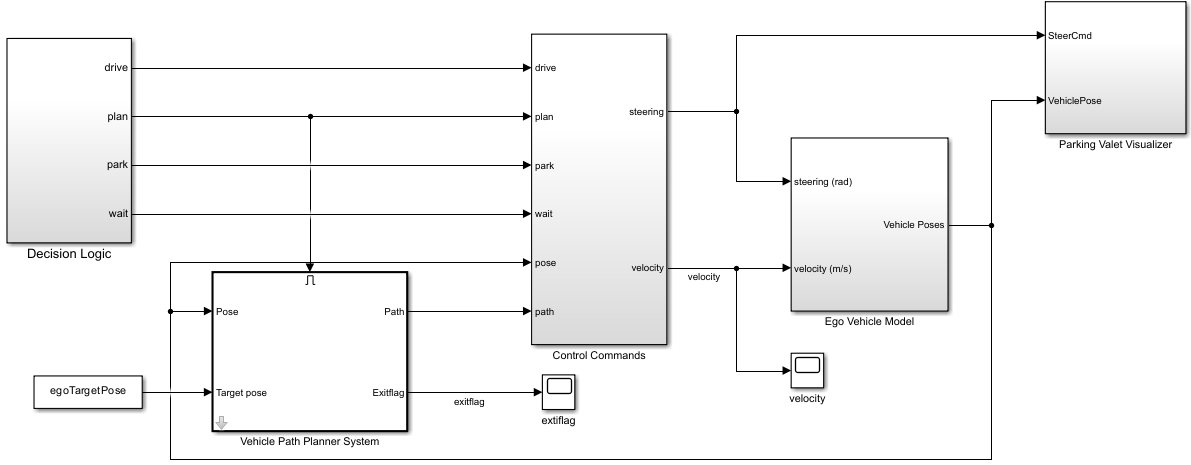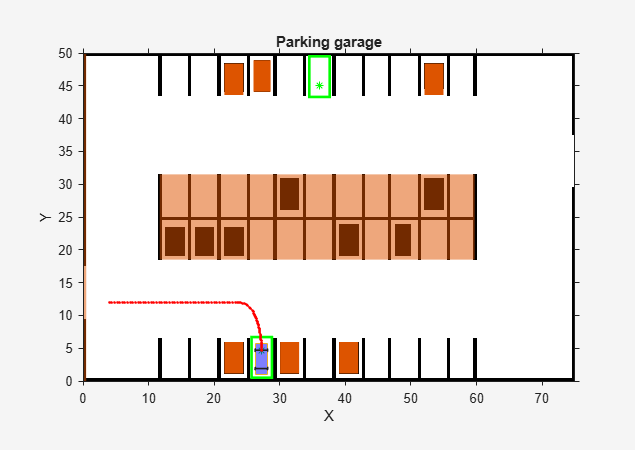### Track Reference Trajectory in Simulink Model

Design an NLMPC controller to track the reference trajectory.

First, set the simulation duration and update the reference trajectory based on the duration.

```Duration = 12; Tsteps = Duration/Ts; Xref = [xRef(2:p+1,:);repmat(xRef(end,:),Tsteps-p,1)];```

Create an NLMPC controller with a tracking prediction horizon (`pTracking`) of `10`.

```pTracking = 10; nlobjTracking = createMPCForTrackingParkingValet(pTracking,Xref);```

Open the Simulink model.

```mdl = 'mpcAutoParkingValet'; open_system(mdl)```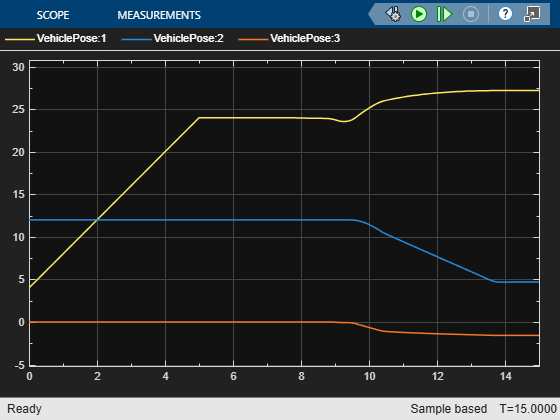Close the animation plots before running the simulation.

```f = findobj('Name','Automated Parking Valet'); close(f)```

Simulate the model.

`sim(mdl)`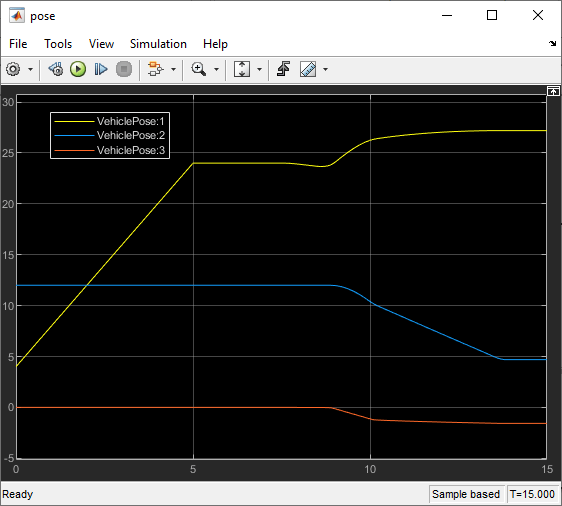```ans = Simulink.SimulationOutput: tout: [126x1 double] SimulationMetadata: [1x1 Simulink.SimulationMetadata] ErrorMessage: [0x0 char] ```

The animation shows that the ego vehicle parks at the target pose successfully without any obstacle collisions. You can also view the ego vehicle and pose trajectories using the Ego Vehicle Pose and Controls scopes.

### Conclusion

This example shows how to generate a reference trajectory and track the trajectory for parking valet using nonlinear model predictive control. The controller navigates the ego vehicle to the target parking spot without colliding with any obstacles.

```mpcverbosity('on'); bdclose(mdl) f = findobj('Name','Automated Parking Valet'); close(f)```

## Support

#### Implementing an Adaptive Cruise Controller with Simulink

Download technical paper